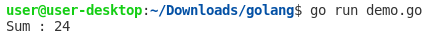## Posts

Showing posts with the label Array

### Java - How to create an ArrayList from an Array?

In this section, we will show you how to create an ArrayList from an Array .  Following ways can be used for converting Array to  ArrayList : By using Arrays.asList() method By using Collections.addAll() method By adding each element of the Array to ArrayList explicitly Example 1: By using Arrays.asList() method  Using asList() method from an Arrays class will convert the given Array to an ArrayList . import java.util.ArrayList ; import java.util.Arrays ; public class Main { public static void main ( String []args) { String [] strArray = { "Java" , "Kotlin" , "C" , "Go" , "Python" }; ArrayList < String > list = new ArrayList< String >( Arrays . asList ( strArray )); System . out .println( list ); } } Console Output: [Java, Kotlin, C, Go, Python] Example 2: By using Collections.addAll() method Using addAll() method from the Collections class will convert

### Java - Program to Find Largest Element in an Array

In this section, we will  show you how to find the largest element in an Array. Using Iterative Method Using  Arrays.sort()  Method 1. Using Iterative Method public class Main { public static void main ( final String [] args) { final int [] array = { 1 , 4 , 44 , 5 , 66 , 2 }; largestElement ( array ); } private static int largestElement ( final int [] array){ // Initialize maximum element int max = array[ 0 ]; // Traverse array elements from second and // compare every element with current max for ( int i = 1 ; i < array. length ; i ++){ if (array[ i ] > max ){ max = array[ i ]; } } System . out .println( max ); return max ; } } Console Output: 66 2. Using Arrays.sort() Method import java.util.Arrays ; public class Main { public static void main ( final String [] args) { final int [] array = { 1 , 4 , 44 , 5 , 66 , 2 }; final

### Remove duplicates from an array in Java

In this section, we will write a Java program to remove duplicate integer elements in an Array. Method 1: Remove duplicates using temp Array To remove the duplicate element from an array, the array must be in sorted order. If an array is not sorted, you can sort it by calling Arrays.sort(array) method. import java.util.Arrays ; public class Main { private static void removeDuplicates ( int [] a) { Arrays . sort (a); int [] temp = new int [a. length ]; int j = 0 ; for ( int i = 0 ; i < a. length - 1 ; i ++) { if (a[ i ] != a[ i + 1 ]) { temp [ j ++] = a[ i ]; } } temp [ j ++] = a[a. length - 1 ]; for ( int i = 0 ; i < j ; i ++) { a[ i ] = temp [ i ]; } for ( int i = 0 ; i < j ; i ++) { System . out .println( temp [ i ]); } } public static void main ( String []args) { int [] a = { 6 , 1 , 1 , 2 , 3 , 4 , 5 , 5

### How to find the sum of an Array/Slice of numbers in Go LanguageGiven an  Array  and  Slice  of Integers, Write a Go Language Program to find the sum of the elements of the  Array  and Slice . Note: Once an  Array  has allocated its size, the size can no longer be transmuted.  A  Slice  is a variable-length version of an  Array , providing more flexibility for developers. A range clause provides a way to iterate over an  Array   or  Slice. Example 1: Program to find the sum of the elements of an Array using for loop range package main import ( "fmt" ) func Sum(numb [ 6 ] int ) int { output := 0 for _, n := range numb { output += n } return output } func main() { num := [ 6 ] int { 5 , 1 , 7 , 2 , 3 , 6 } fmt.Println( "Sum :" , Sum(num)) } Output: Example 2: Program to find the sum of the elements of a Slice using for loop range package main import ( "fmt" ) func Sum(numb [] int ) int { output := 0 for _, n := range numb { output += n } return